# Algebra 1 : Decimals and Percentage

## Example Questions

### Example Question #2991 : Algebra 1

Find the decimal equivalent of the following percent: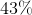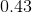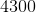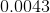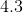Explanation:

In order to find the decimal equivalent for a percent, all we have to do is divide our percent by 100. We can also move the decimal point to the left two spaces.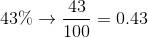### Example Question #62 : Decimals And Percentage

Find the decimal equivalent of the following percent: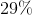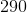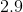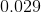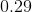Explanation:

In order to find the decimal equivalent for a percent, all we have to do is divide our percent by 100. We can also move the decimal point to the left two spaces.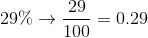### Example Question #63 : Decimals And Percentage

Find the decimal equivalent of the following percent: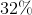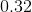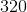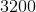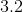Explanation:

In order to find the decimal equivalent for a percent, all we have to do is divide our percent by 100. We can also move the decimal point to the left two spaces.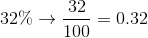### Example Question #61 : Decimals And Percentage

Find the decimal equivalent of the following percent: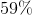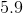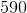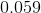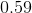Explanation:

In order to find the decimal equivalent for a percent, all we have to do is divide our percent by 100. We can also move the decimal point to the left two spaces.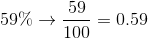### Example Question #2991 : Algebra 1

Find the decimal equivalent for the following percent: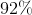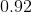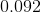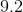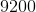Explanation:

In order to find the decimal equivalent for a percent, all we have to do is divide our percent by 100. We can also move the decimal point to the left two spaces.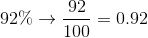### Example Question #61 : Decimals And Percentage

Find the decimal equivalent of the following percent: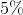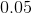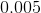Explanation:

In order to find the decimal equivalent for a percent, all we have to do is divide our percent by 100. We can also move the decimal point to the left two spaces.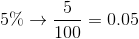### Example Question #61 : Decimals And Percentage

Find the decimal equivalent of the following percent: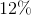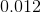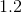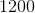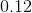Explanation:

In order to find the decimal equivalent for a percent, all we have to do is divide our percent by 100. We can also move the decimal point to the left two spaces.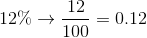### Example Question #63 : Decimals And Percentage

Find the decimal equivalent of the following percent: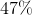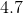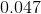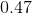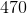Explanation:

In order to find the decimal equivalent for a percent, all we have to do is divide our percent by 100. We can also move the decimal point to the left two spaces.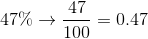### Example Question #61 : Decimals And Percentage

What is the decimal equivalen of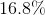?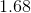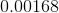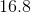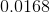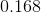Explanation:

To find the decimal equivalent of a percentage, take the percentage and divide it by one-hundred: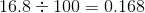### Example Question #64 : Decimals And Percentage

What is the decimal equivalent of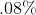?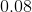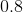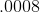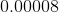When presented with a percentage, we can effectively "move" the decimal point over two spots and arrive at the equivalent decimal. In our example,turns toin decimal form.